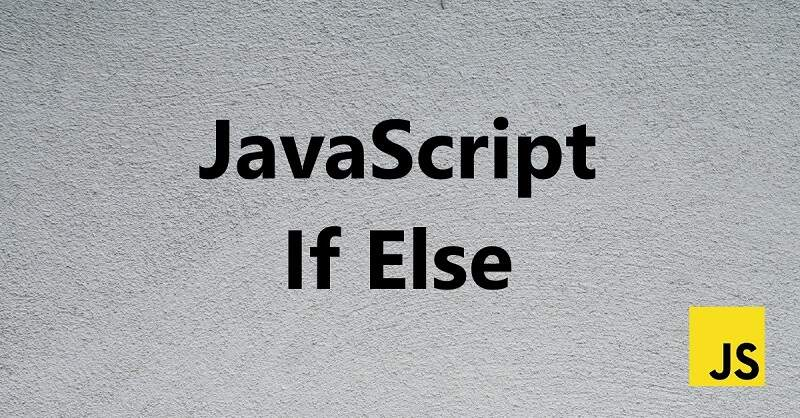# JavaScript If ElseConditional statements are an important part of JavaScript that allows us to perform various actions depending upon the condition. We can use conditional statements to execute a particular piece of code when a certain condition is met.

In this JavaScript tutorial, you will learn about the if else conditional statement.

## Forms of if-else Statement

There are three forms of if else statement, which are listed as follows:

1. if statement
2. if-else statement
3. If-else if statement

### 1. JavaScript If Statement

The JavaScript if statement executes the block of code inside the main body in case the specified condition comes out to be true.

Syntax:

if (condition) {

// Some code to be executed if the condition is evaluated to true.

}

Example:

```var x = 5;

if (x<10) {

console.log (“x is smaller than 10”)

};```

### 2. JavaScript If-else Statement

When it comes to the if else statement, the code under the if statement gets executed in case the specified condition is true. On the other hand, in case the specified condition comes out to be false, the code under the else statement gets executed.

Syntax:

if (condition) {

// Code to be executed if the condition is evaluated to true.

}

else {

// Code to be executed if the condition is evaluated to false.

}

Example:

```var x = 5;

if (x<10) {

console.log (“x is smaller than 10”);

}

else {

console.log (“x is greater than or equal to 10”);

}```

### 3. JavaScript If-else if statement

The if-else if statement makes it possible to specify more than one condition and execute code accordingly.

Syntax:

if (condition 1) {

// Code to be executed if the condition 1 is evaluated to true.

}

else if (condition 2) {

// Code to be executed if the condition 2 is evaluated to true.

}

else if (condition 3) {

// Code to be executed if the condition 3 is evaluated to true.

}

else {

// Code to be executed if the condition 1 is evaluated to false.

}

Example:

```var x = 5;

if (x<10) {

console.log (“x is smaller than 10”);

}

else if (x ==10) {

console.log (“x is equal to 10”)

}

else {

console.log (“x is greater than 10”);

}```

## To Sum it Up

Learning how JavaScript if else statement works is essential if you want to become proficient in writing JavaScript code. The if else statement and its different forms allow you to execute a particular block of code if a certain condition becomes true or false.